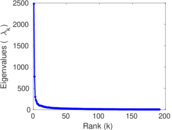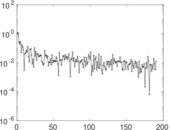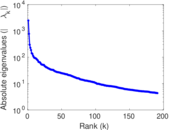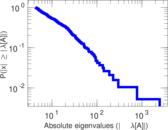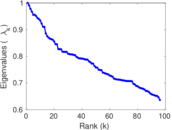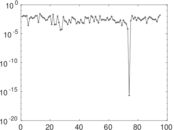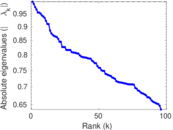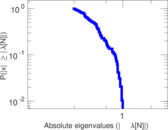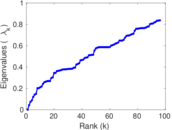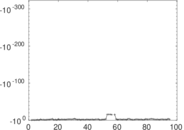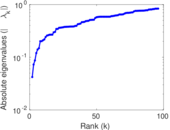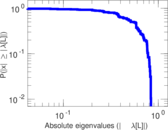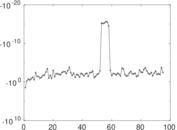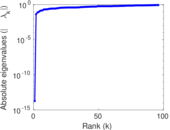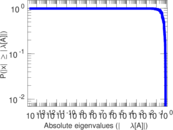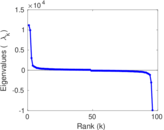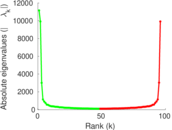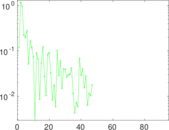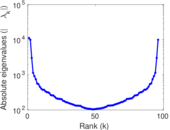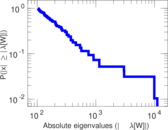# Wikivoyage edits (sv)

This is the bipartite edit network of the Swedish Wikivoyage. It contains users and pages from the Swedish Wikivoyage, connected by edit events. Each edge represents an edit. The dataset includes the timestamp of each edit.

 Code `vsv` Internal name `edit-svwikivoyage` Name Wikivoyage edits (sv) Data source http://dumps.wikimedia.org/ AvailabilityDataset is available for download Consistency checkDataset passed all tests Category Authorship network Dataset timestamp 2017-10-20 Node meaning User, article Edge meaning Edit Network formatBipartite, undirected Edge typeUnweighted, multiple edges Temporal dataEdges are annotated with timestamps

## Statistics

 Size n = 11,151 Left size n1 = 748 Right size n2 = 10,403 Volume m = 65,410 Unique edge count m̿ = 27,511 Wedge count s = 21,383,567 Claw count z = 21,614,599,387 Cross count x = 21,088,263,378,154 Square count q = 17,051,705 4-Tour count T4 = 222,064,018 Maximum degree dmax = 15,402 Maximum left degree d1max = 15,402 Maximum right degree d2max = 2,479 Average degree d = 11.731 7 Average left degree d1 = 87.446 5 Average right degree d2 = 6.287 61 Fill p = 0.003 535 46 Average edge multiplicity m̃ = 2.377 59 Size of LCC N = 10,922 Diameter δ = 11 50-Percentile effective diameter δ0.5 = 3.281 89 90-Percentile effective diameter δ0.9 = 3.938 34 Median distance δM = 4 Mean distance δm = 3.495 81 Gini coefficient G = 0.847 715 Balanced inequality ratio P = 0.138 993 Left balanced inequality ratio P1 = 0.066 809 4 Right balanced inequality ratio P2 = 0.199 159 Relative edge distribution entropy Her = 0.733 330 Power law exponent γ = 2.991 89 Tail power law exponent γt = 2.081 00 Tail power law exponent with p γ3 = 2.081 00 p-value p = 0.000 00 Left tail power law exponent with p γ3,1 = 1.731 00 Left p-value p1 = 0.004 000 00 Right tail power law exponent with p γ3,2 = 2.121 00 Right p-value p2 = 0.000 00 Degree assortativity ρ = −0.332 302 Degree assortativity p-value pρ = 0.000 00 Spectral norm α = 2,479.00 Algebraic connectivity a = 0.042 021 6 Spectral separation |λ1[A] / λ2[A]| = 3.198 18 Controllability C = 9,884 Relative controllability Cr = 0.888 849

## Plots

### Fruchterman–Reingold graph drawing### Degree distribution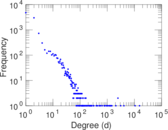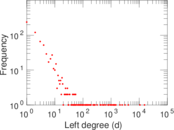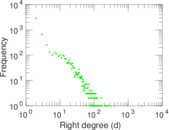### Cumulative degree distribution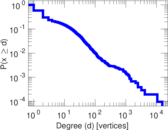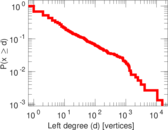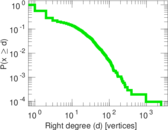### Lorenz curve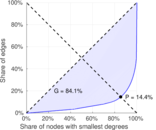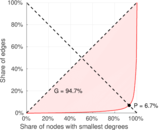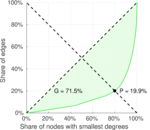### Spectral distribution of the adjacency matrix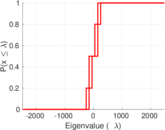### Spectral distribution of the normalized adjacency matrix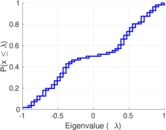### Spectral distribution of the Laplacian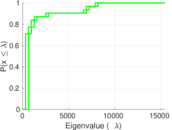### Spectral graph drawing based on the adjacency matrix### Spectral graph drawing based on the Laplacian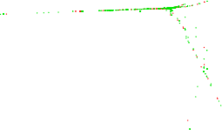### Spectral graph drawing based on the normalized adjacency matrix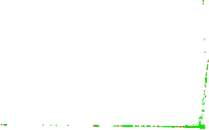### Degree assortativity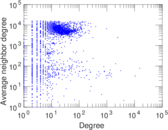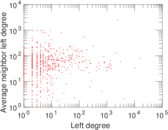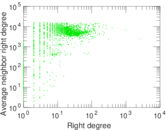### Zipf plot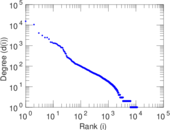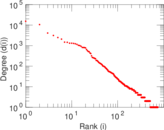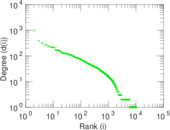### Hop distribution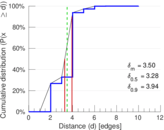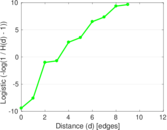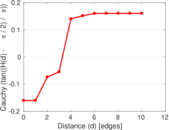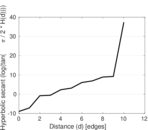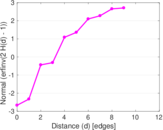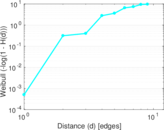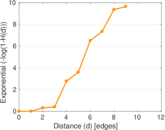### Double Laplacian graph drawing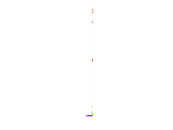### Delaunay graph drawing### Edge weight/multiplicity distribution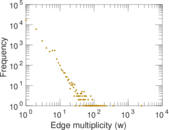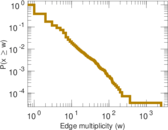### Temporal distribution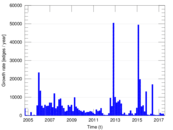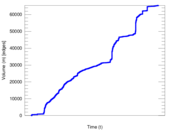### Temporal hop distribution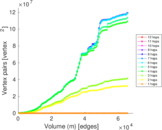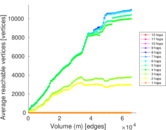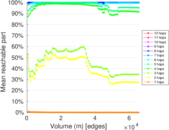### Diameter/density evolution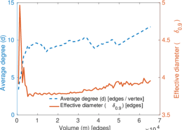### Matrix decompositions plots